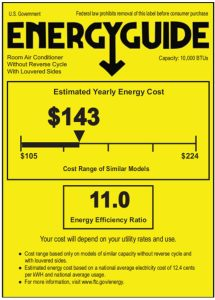Sep 1, 2022

# How Many Volts and Amps Does an Air Conditioner Use?

The wattage of an appliance is determined by its voltage and amperage. You can use the yellow EnergyGuide label on your air conditioning to determine the volts and amps it uses.Using the above label, here’s how you can calculate volts and amps:

1. Calculate the estimated yearly electricity usage by dividing the estimated yearly energy cost (\$143) by the national average electricity cost per kWh (12.4 cents): \$143 / \$0.124/kWh = 1,153 kWh.
2. Translate energy consumption to watt-hours (Wh) by multiplying the label’s kWh by 1,000. This gives you 1,153,000 Wh.
3. Divide 1,153,000 Wh by the number of days in a year (365), which gives you 3,159 Wh per day.
4. Divide 3,159 Wh per day by the number of hours a day (24) to get an average hourly wattage of 132 W.
5. Air conditioners usually use 120-volt outlets. Divide the 132 W by 120 volts to get the amperage for your appliance: 132 W / 120 V = 1.1 amps.

### Watts, amps, voltage, and more: what do they mean?

There are a lot of terms you can use to describe how electricity flows and is used by appliances. We’ve already mentioned most of them – here are a few definitions to keep things straight:

• Volts (V): volts (short for voltage) measure electrical pressure differences. Voltage is the speed of electricity passing through a circuit.
• Amps (A): amps (short for amperes) measure electrical current. Amps are the amount of electrons (which make up electricity) flowing through a circuit.
• Watts (W) and kilowatts (kW): multiplying volts x amps get you watts (or wattage). Watts are the rate of electricity consumption. A kilowatt is just 1,000 watts.
• Kilowatt-hours (kWh) are how your electric bill measures your energy usage. Simply put, kilowatt-hours are electricity consumption over time.

You can think of all these terms as water flowing through a pipe. Voltage is the water pressure, amps are the amount of water flowing past any point, and wattage is the overall rate of water flow through the pipe.

### How much does it cost to power an air conditioner?

When you get your monthly electric bill, you only see the total amount you are charged, not how much each appliance contributes to your final bill. Based on an average running wattage of 2,275 W for central air conditioners (amounting to 8,304 kWh/year) and 950 W for window air conditioners (amounting to 2,256 kWh/year), and using state average electricity rates.

Note: average electricity rates are based on October 2021 data from the U.S. Energy Information Administration (EIA).

### Calculate how much energy your air conditioner uses

Remember that yellow Energy Saver sticker we mentioned above? Suppose you want to know how much electricity your air conditioner uses (or is supposed to use). Take the estimated yearly electricity use in kWh. In that case, this is probably your best bet for an accurate number. Multiply this number by the average electricity rate in your area to estimate how much you spend to power your air conditioner each year. For an estimated monthly cost, divide the estimated yearly cost by 12.

### Heat pumps are an efficient alternative to traditional AC

Air source heat pumps are an efficient electric heating and cooling option for your home or business and are a great alternative to traditional central or window AC. You may have heard of “mini-splits” or “ductless AC” before – that’s just another way to talk about heat pumps. Heat pumps are usually far more efficient (and therefore less expensive) in cold climates than air conditioning.

## BRAND PARTNERS & ASSOCIATIONS